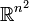# General linear group over reals

## Definition

The general linear group over reals of degree$n$, denoted$GL(n,\R)$ or$GL_n(\R)$, is defined as the general linear group of degree$n$ over the field of real numbers$\R$.

Some of the properties of these general linear groups generalize to general linear groups over fields that resemble the reals in one or more important respect: for instance, formally real fields, totally real fields, ordered fields, Pythagorean fields, and quadratically closed fields.

### Structures

Each group$GL(n,\R)$ can be thought of in any of the following ways:

## Arithmetic functions

Function Value Similar groups Explanation
dimension of an algebraic group$n^2$ The group is a Zariski open subset of the matrix algebra (which can be identified with$\R^{n^2}$), defined as the set of elements of nonzero determinant. Note that the determinant map is a polynomial map.
dimension of a real Lie group$n^2$ The group is an open subset of the matrix algebra (which can be identified with$\R^{n^2}$), defined as the set of elements of nonzero determinant. Note that the determinant map is jointly continuous

## Group properties

### Abstract group properties

Property Satisfied? Explanation
abelian group No except for$n = 1$ Follows from center of general linear group is group of scalar matrices over center
nilpotent group No except for$n = 1$ Follows from special linear group is quasisimple
solvable group No except for$n = 1$ Follows from special linear group is quasisimple
simple group No Has a proper nontrivial center, also has normal subgroup$SL(2,\R)$.
almost simple group No Has a nontrivial center.
quasisimple group No Not a perfect group; has a nontrivial homomorphism to an abelian group, namely the determinant map
almost quasisimple group Yes Follows from special linear group is quasisimple

### Topological/Lie group properties

The topology here is the subspace topology from the Euclidean topology on the set of all matrices, which is identified with the Euclidean space$\R^4$.

Property Satisfied? Explanation
connected topological group No the matrices with positive determinant form one connected component. The matrices with negative determinant form the other connected component.
compact group No The determinant map is continuous and surjective to the non-compact set of nonzero reals.